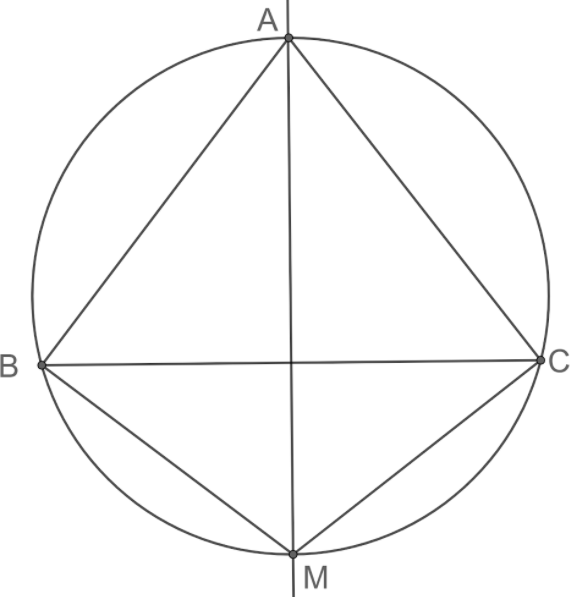‘I’ is the perpendicular bisector of side BC of △ABC meeting the circumference of the triangle of M opposite to A then

# ‘I’ is the perpendicular bisector of side BC of △ABC meeting the circumference of the triangle of M opposite to A then

1. A
∠MBC=∠MCB2. B
∠BCM=∠BAM
3. C
∠CMB=∠CAM
4. D
∠BAM=∠CAM

Fill Out the Form for Expert Academic Guidance!l

+91

Live ClassesBooksTest SeriesSelf Learning

Verify OTP Code (required)

### Solution:

An arc in a circle subtends equal angles anywhere on the circumference.
BM is the chord.
BCM=∠BAM
Hence, option 2) is correct.
1) ∠MBC=∠MCB
MBC is subtended at the arc MC and the angle ∠MCB is subtended at the arc BM.
For the property, the angles should be subtended at the same arc.
Therefore, option 1) is incorrect.
3) ∠CMB=∠CAM
CMB is subtended at the arc BC and the angle ∠CAM is subtended at the arc CM.
For the property, the angles should be subtended at the same arc.
Therefore, option 3) is incorrect.
4) ∠BAM=∠CAM
BAM is subtended at the arc BM and the angle ∠CAM is subtended at the arc CM.
For the property, the angles should be subtended at the same arc.
Therefore, option 4) is incorrect.
So, Option 2 is correct.

## Related content

 Area of Square Area of Isosceles Triangle Pythagoras Theorem Triangle Formula Perimeter of Triangle Formula Area Formulae Volume of Cone Formula Matrices and Determinants_mathematics Critical Points Solved Examples Type of relations_mathematics+91

Live ClassesBooksTest SeriesSelf Learning

Verify OTP Code (required)